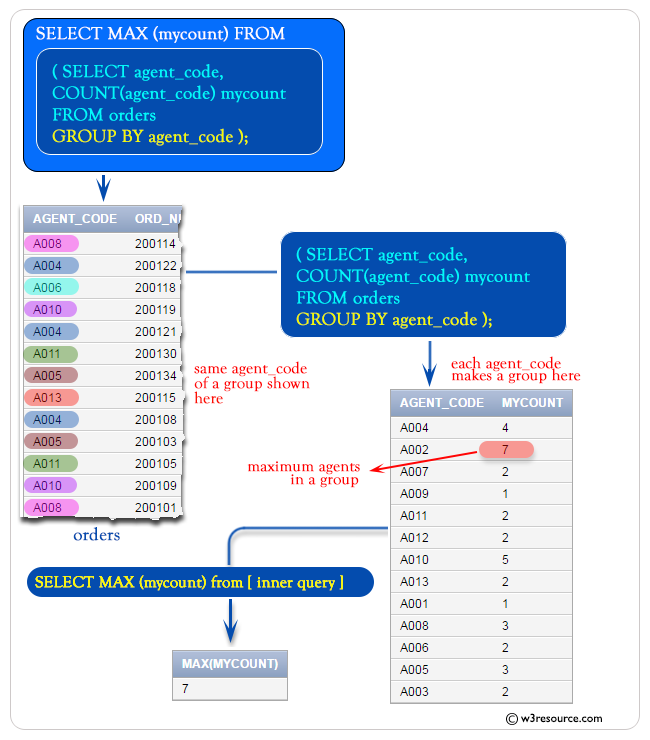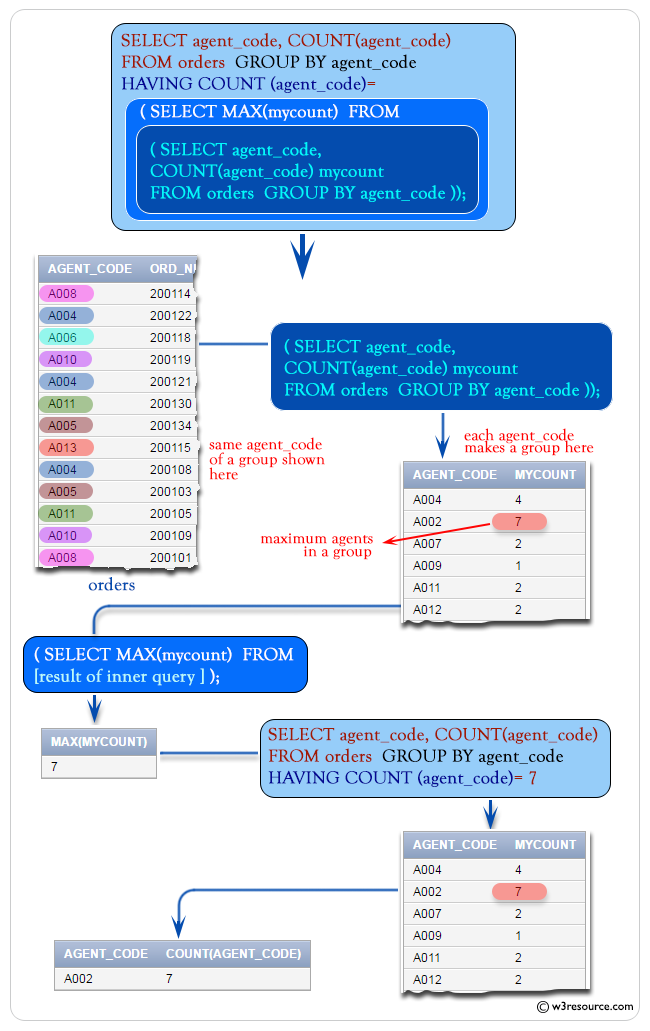# SQL MAX() with COUNT()

## MAX() with Count function

In this part, you will see the usage of SQL COUNT() along with the SQL MAX().

Example:

To get the maximum number of agents as column alias 'mycount' from the 'orders' table with the following condition -

1. 'agent_code' should be in a group,

the following SQL statement can be used :

``````SELECT MAX (mycount)
FROM (SELECT agent_code,COUNT(agent_code) mycount
FROM orders
GROUP BY agent_code);``````

Sample table: orders

Output:

```MAX(MYCOUNT)
------------
7
```

Pictorial Presentation :## SQL MAX() and COUNT() with HAVING

To get data of 'agent_code', and number of agents for each group of 'agent_code' from the orders table with the following conditions -

'agent_code' for a group will be equal to the result of an outer query [SELECT MAX(agent_code).......] with following condition -

the outer query produce the maximum number of agents mentioned as 'mycount' from an inner query [SELECT agent_code,
COUNT(agent_code) mycount FROM orders GROUP BY agent_code] with following condition -

the inner query produced the data 'agent_code' number of agents as column alias 'mycount' from the 'orders' table with the following condition -

'agent_code' should be in a group,

the following SQL statement can be used :

``````SELECT agent_code, COUNT(agent_code)
FROM orders  GROUP BY agent_code
HAVING COUNT (agent_code)=(
SELECT MAX(mycount)
FROM (
SELECT agent_code, COUNT(agent_code) mycount
FROM orders
GROUP BY agent_code));``````

Sample table: orders

Output:

```AGENT_CODE COUNT(AGENT_CODE)
---------- -----------------
A002                       7
```Note: Outputs of the said SQL statement shown here is taken by using Oracle Database 10g Express Edition

Here is a slide presentation of all aggregate functions.

Check out our 1000+ SQL Exercises with solution and explanation to improve your skills.

Previous: Max Date
Next: Min function

﻿

## SQL: Tips of the Day

MySQL select 10 random rows from 600K rows fast:

```SELECT name
FROM random AS r1 JOIN
(SELECT CEIL(RAND() *
(SELECT MAX(id)
FROM random)) AS id)
AS r2
WHERE r1.id >= r2.id
ORDER BY r1.id ASC
LIMIT 1
```

Ref: https://bit.ly/3GdCTM3

We are closing our Disqus commenting system for some maintenanace issues. You may write to us at reach[at]yahoo[dot]com or visit us at Facebook